Скачать презентацию Algebra I 1 1 Evaluate Expressions Question

3471906d4177324a063e8e5439b9b540.ppt

• Количество слайдов: 14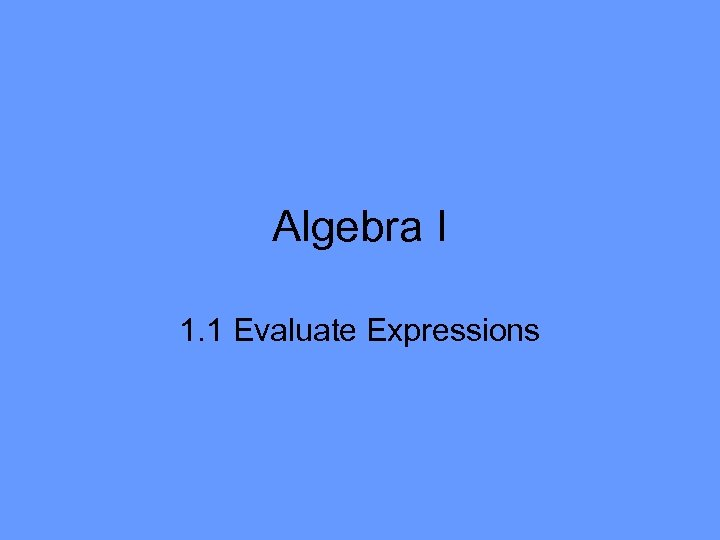Algebra I 1. 1 Evaluate Expressions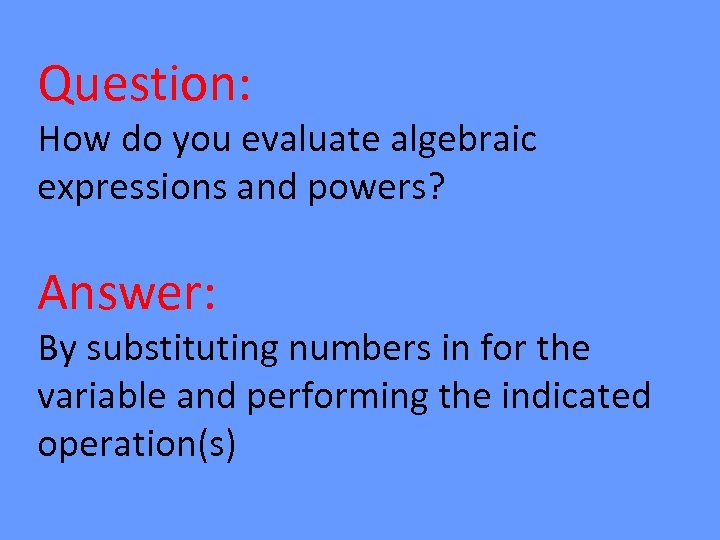Question: How do you evaluate algebraic expressions and powers? Answer: By substituting numbers in for the variable and performing the indicated operation(s)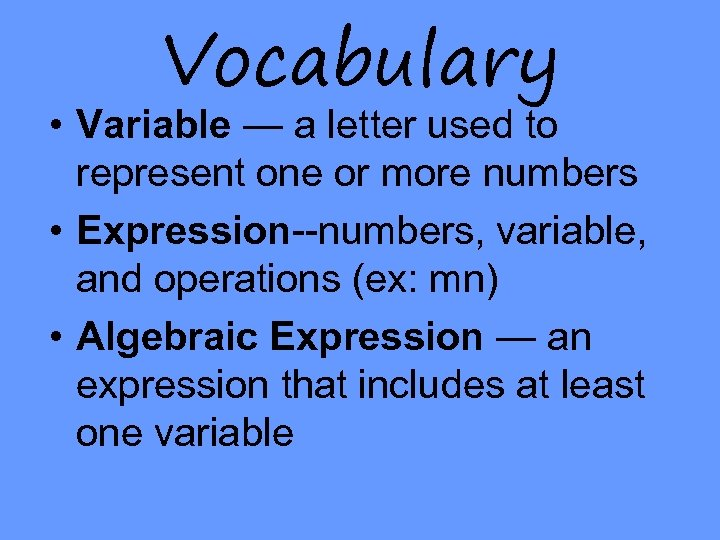Vocabulary • Variable — a letter used to represent one or more numbers • Expression--numbers, variable, and operations (ex: mn) • Algebraic Expression — an expression that includes at least one variable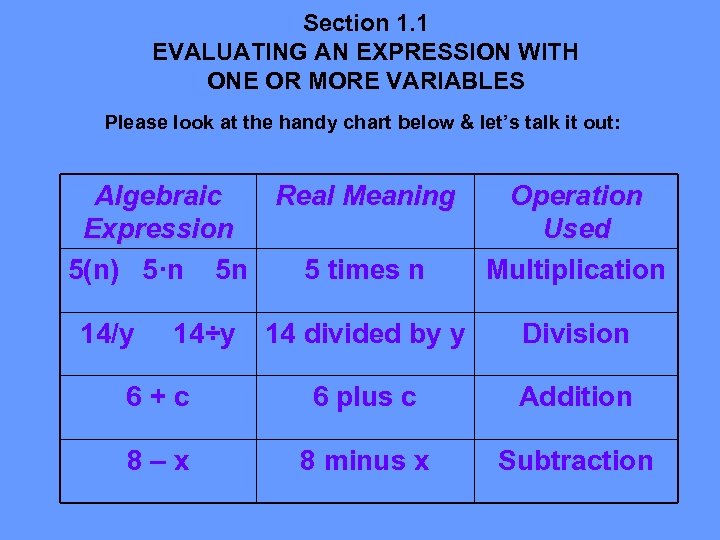Section 1. 1 EVALUATING AN EXPRESSION WITH ONE OR MORE VARIABLES Please look at the handy chart below & let’s talk it out: Algebraic Real Meaning Expression 5(n) 5·n 5 n 5 times n 14/y 14÷y Operation Used Multiplication 14 divided by y Division 6+c 6 plus c Addition 8–x 8 minus x Subtraction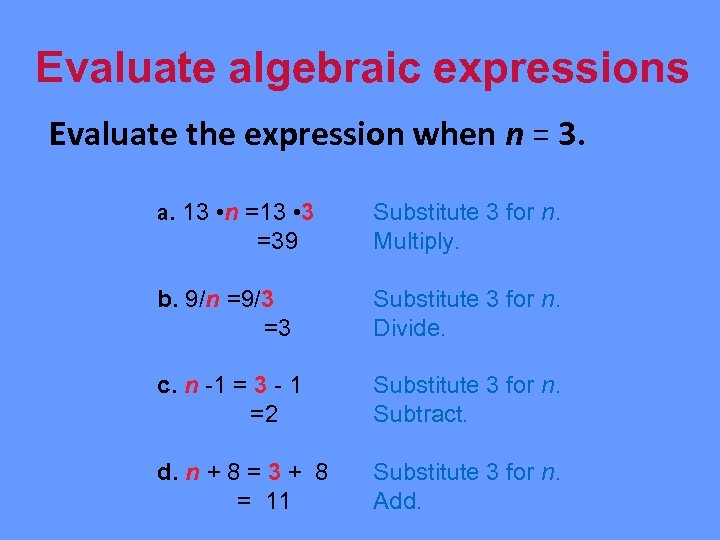Evaluate algebraic expressions Evaluate the expression when n = 3. a. 13 • n =13 • 3 =39 Substitute 3 for n. Multiply. b. 9/n =9/3 =3 Substitute 3 for n. Divide. c. n -1 = 3 - 1 =2 Substitute 3 for n. Subtract. d. n + 8 = 3 + 8 = 11 Substitute 3 for n. Add.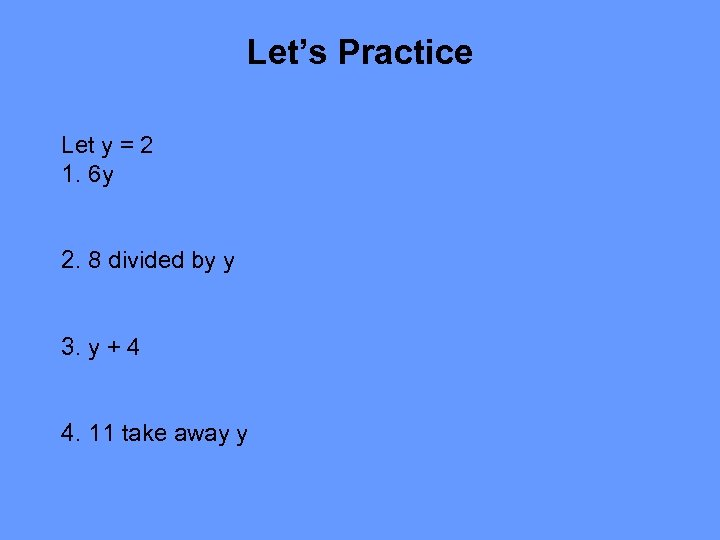Let’s Practice Let y = 2 1. 6 y 2. 8 divided by y 3. y + 4 4. 11 take away y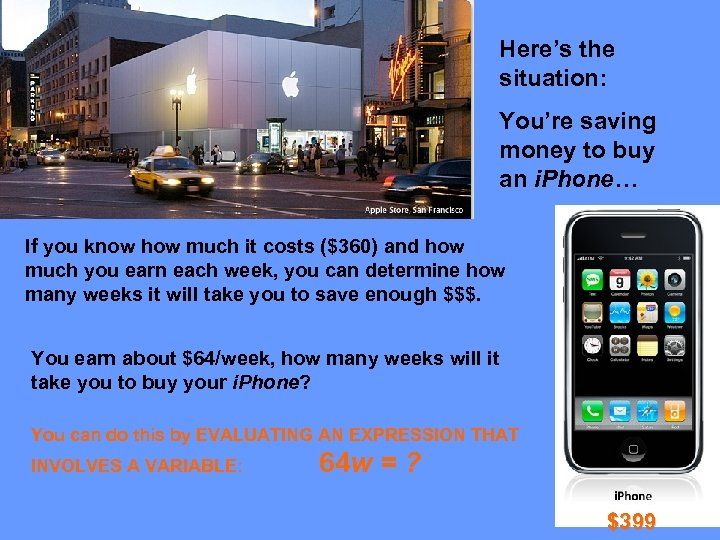Here’s the situation: You’re saving money to buy an i. Phone… If you know how much it costs (\$360) and how much you earn each week, you can determine how many weeks it will take you to save enough \$\$\$. You earn about \$64/week, how many weeks will it take you to buy your i. Phone? You can do this by EVALUATING AN EXPRESSION THAT INVOLVES A VARIABLE: 64 w = ? \$399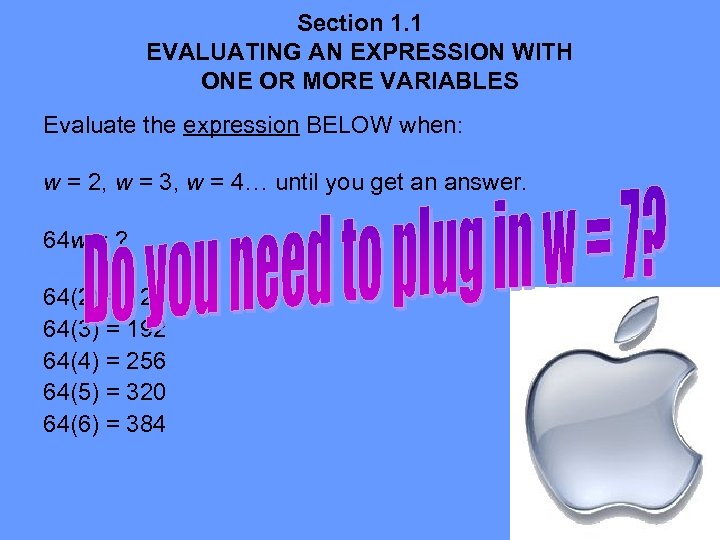Section 1. 1 EVALUATING AN EXPRESSION WITH ONE OR MORE VARIABLES Evaluate the expression BELOW when: w = 2, w = 3, w = 4… until you get an answer. 64 w = ? 64(2) = 128 64(3) = 192 64(4) = 256 64(5) = 320 64(6) = 384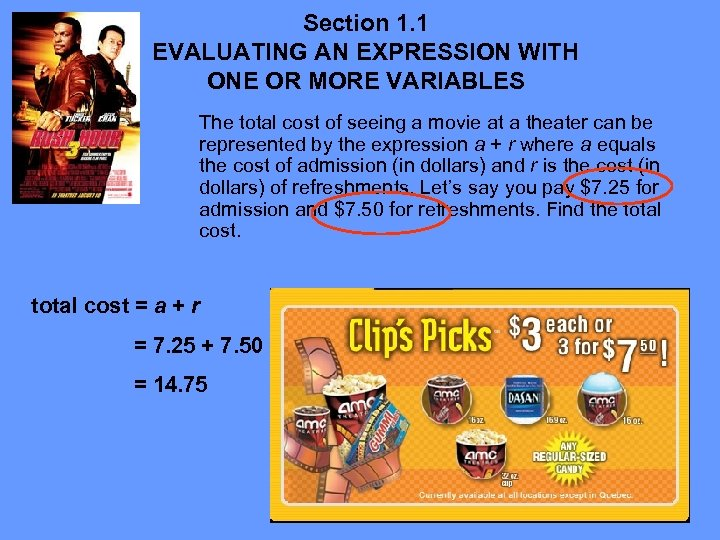Section 1. 1 EVALUATING AN EXPRESSION WITH ONE OR MORE VARIABLES The total cost of seeing a movie at a theater can be represented by the expression a + r where a equals the cost of admission (in dollars) and r is the cost (in dollars) of refreshments. Let’s say you pay \$7. 25 for admission and \$7. 50 for refreshments. Find the total cost = a + r = 7. 25 + 7. 50 = 14. 75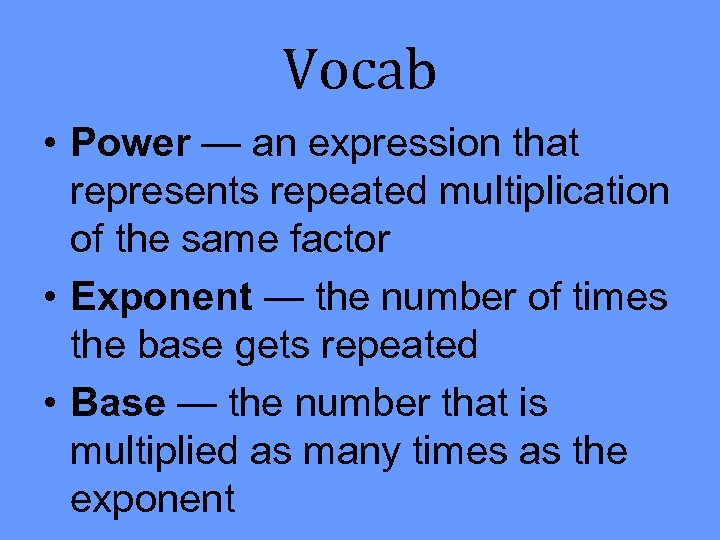Vocab • Power — an expression that represents repeated multiplication of the same factor • Exponent — the number of times the base gets repeated • Base — the number that is multiplied as many times as the exponent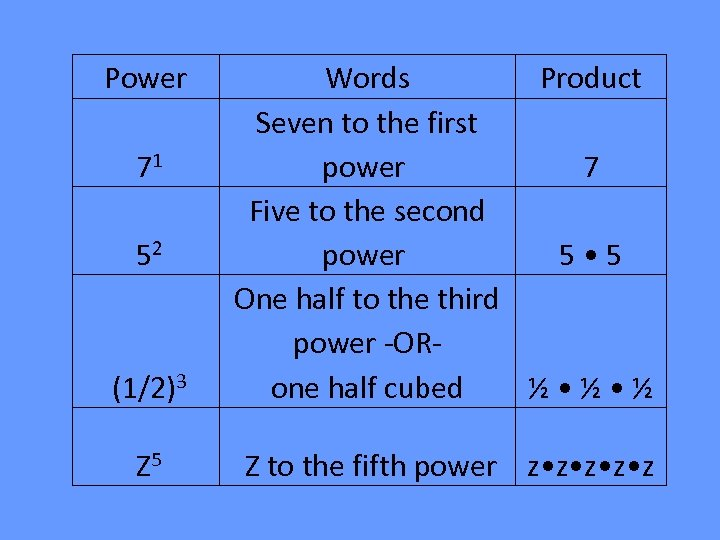Power (1/2)3 Words Product Seven to the first power 7 Five to the second power 5 • 5 One half to the third power -OR½ • ½ one half cubed Z 5 Z to the fifth power z • z • z 71 52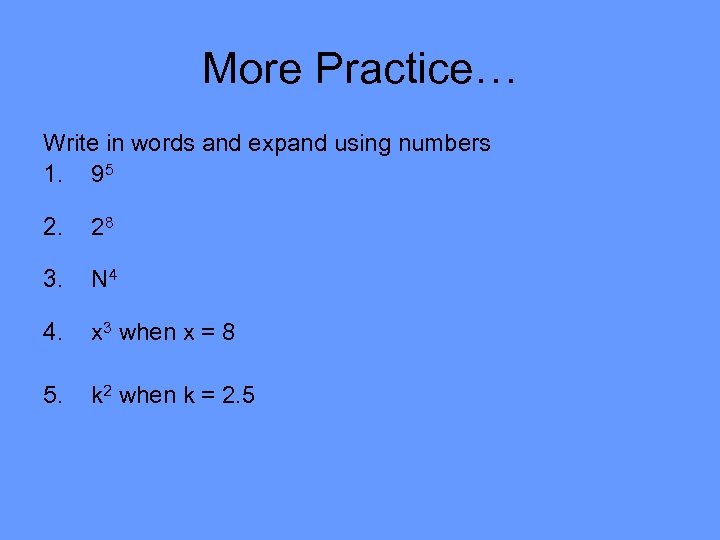More Practice… Write in words and expand using numbers 1. 95 2. 28 3. N 4 4. x 3 when x = 8 5. k 2 when k = 2. 5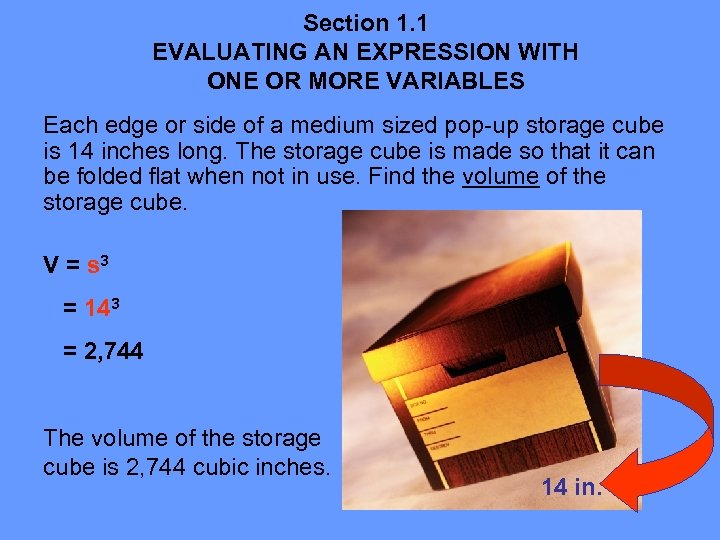Section 1. 1 EVALUATING AN EXPRESSION WITH ONE OR MORE VARIABLES Each edge or side of a medium sized pop-up storage cube is 14 inches long. The storage cube is made so that it can be folded flat when not in use. Find the volume of the storage cube. V = s 3 = 143 = 2, 744 The volume of the storage cube is 2, 744 cubic inches. 14 in.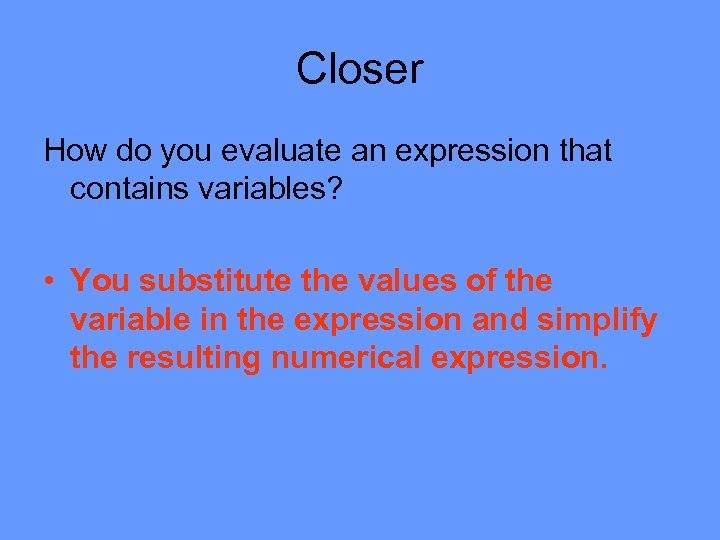Closer How do you evaluate an expression that contains variables? • You substitute the values of the variable in the expression and simplify the resulting numerical expression.